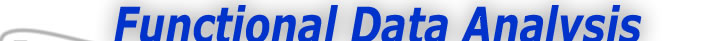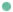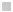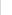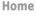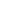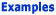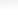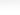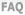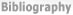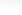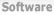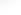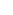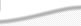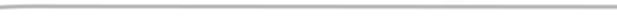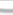BasisFunctions The Basics of Basis Functions -What is a Basis Function -How do I know what basis system to use? Goods Growth Weather
 The Basics of Basis Functions Previous - 1 - 2 - Next Expertise: Beginner How do I know what basis system to use? Most data-fitting now uses Fourier series for periodic or near periodic data, such as weather data, some types of economic data, and so on. Otherwise, when the data are not periodic, we use splines. Why? Well, consider what we want from a set of functional building blocks. Fast computation of individual basis functions: We may need thousands of basis functions in some situations, and being able to evaluate øk(t) fast is crucial. Which is why polynomials were so important in the days of hand calculation. Modern methods of computation also permit the rapid evaluation of sines, cosines, and other transcendental functions. Flexibility: Many curves have features involving sharp curvature at specific locations. Polynomials can track such features only by using a large number of basis functions, but splines are much better at fitting highly curvy data. Fourier series aren't great at capturing sharp changes, but wavelets are terrific at this. Fast computation of coefficients ak: Coefficients are calculated by solving a system of linear equations, with one equation for each basis function. This isn't hard if there is under a hundred of these, but if thousands are required, only basis functions systems that imply special structures for these equations will serve. Again, splines are excellent, but polynomials, Fourier series and wavelets will only work for relatively specialized situations, such as equally spaced values of t. Differentiability as required: Derivatives play many roles in data modelling, and we will want basis functions that have well-behaved derivatives up to whatever order that we require. Fourier series certainly work here, as do splines. But polynomials fall down in the sense that their derivatives exhibit progressively more simple behavior the higher the order, and the real world tends not to behave like this. Special constraints: Some functions can only be positive, others may be required to be strictly increasing, and we may want functions to become more and more like straight lines for extreme values of t. These constraints also need to be built into our basis systems. A common example is periodicity, and we have already noted that Fourier series, as well as wavelets, are the basis systems of choice in this case. What else do I need to know? Using polynomials or Fourier series is easy. In either case, you just decide how many basis functions, Κ, you will need to use to give yourself enough fitting power, and nearly any statistical package, numerical computation software, or high level programming language will set up the necessary linear equations defining the ak's and solve them. In the Fourier case, you will also need to know the base frequency or period, defined by ω and the rule that rule that the period P is equal to 2π/ω, or ω = 2π/P. Your next task is to learn how to work with splines, since they are the basis of choice for most problems involving non-periodic data. Have a look, therefore, at the web pages describing splines. Previous - 1 - 2 - Next